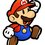# Hard Math

I remember some math questions from the math Olympiads, so can you do better than I can? Good luck!

1) a-squared +b-squared=2018-2a, what is a and b?

2) What is the last non-0 digit of 50!

3a) a and b are positive integers, where a= the sum of all the digits of a and b, and b= the product of all the digits of a and b. Find all pairs that satisfy this equation, under 100.

3b) FInd all pairs under 1000, and one of the numbers has to be over 99.

Really, I only got an answer for 2 (probably wrong), and nothing for the rest. Please tell me what you got, and please note, I might have remembered this wrong. But, it should still be right. How did you go? :)Note by Caleb Kum
1 year, 10 months ago

This discussion board is a place to discuss our Daily Challenges and the math and science related to those challenges. Explanations are more than just a solution — they should explain the steps and thinking strategies that you used to obtain the solution. Comments should further the discussion of math and science.

When posting on Brilliant:

• Use the emojis to react to an explanation, whether you're congratulating a job well done , or just really confused .
• Ask specific questions about the challenge or the steps in somebody's explanation. Well-posed questions can add a lot to the discussion, but posting "I don't understand!" doesn't help anyone.
• Try to contribute something new to the discussion, whether it is an extension, generalization or other idea related to the challenge.

MarkdownAppears as
*italics* or _italics_ italics
**bold** or __bold__ bold
- bulleted- list
• bulleted
• list
1. numbered2. list
1. numbered
2. list
Note: you must add a full line of space before and after lists for them to show up correctly
paragraph 1paragraph 2

paragraph 1

paragraph 2

[example link](https://brilliant.org)example link
> This is a quote
This is a quote
    # I indented these lines
# 4 spaces, and now they show
# up as a code block.

print "hello world"
# I indented these lines
# 4 spaces, and now they show
# up as a code block.

print "hello world"
MathAppears as
Remember to wrap math in $$ ... $$ or $ ... $ to ensure proper formatting.
2 \times 3 $2 \times 3$
2^{34} $2^{34}$
a_{i-1} $a_{i-1}$
\frac{2}{3} $\frac{2}{3}$
\sqrt{2} $\sqrt{2}$
\sum_{i=1}^3 $\sum_{i=1}^3$
\sin \theta $\sin \theta$
\boxed{123} $\boxed{123}$

Sort by:

Q1) The answer is that there are no possible INTEGRAL a and b satisfying the equation..................otherwise there are infinitely many pairs of a and b..........

- 1 year, 10 months ago

What I found that it may seem like that, but when I did it, it would always be short. So, (Idk if this is right) there should be only one answer.

- 1 year, 10 months ago

I rechecked my calculations and found no error..........Check again..........There are no Integral a and b satisfying the condition...........If you have found a solution, then tell me the answer....... :)

- 1 year, 10 months ago

Ok, I'll take your word for it. Hey, anyone, can YOU find an answer? (I can't be stuffed to do so).

- 1 year, 10 months ago